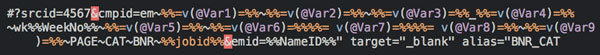Hi

Is there a way to check a specify set of code in link tracking/queries?

Would like to check ALL the required `@Var` are in the Query and nothing has been accidentally trimmed off, when hand coding it.

``````#?srcid=4567&cmpid=em~%%=v(@Var1)=%%~%%=v(@Var2)=%%~%%=v(@Var3)=%%_%%=v(@Var4)=%%~wk%%WeekNo%%~%%=v(@Var5)=%%~%%=v(@Var6)=%%%%= v(@Var7)=%%%%= v(@Var8)=%%~%%=v(@Var9)=%%~PAGE~CAT~BNR~%%jobid%%&emid=%%NameID%%" target="_blank" alias="BNR_CAT
``````Open to suggestion of when or where this happens, thinking ideally it would happen in the browser when testing locally the code.

*Maybe it happens as an Addon or a Javascript script locally or even sent-time through the ESP?

Appreciate any help on this.

Thanks